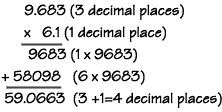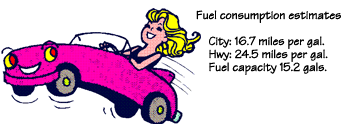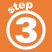Home    |    Teacher    |    Parents    |    Glossary    |    About UsFind the product of 9.683 x 6.1=?1. Line up the numbers on the right,
2. multiply each digit in the top number by each digit in the bottom number (like whole numbers),
4. and mark off decimal places equal to the sum of the decimal places in the numbers being multiplied.

Try this word problem. You'll use your estimating skills as well as your multiplying skills.Jackie just bought a new car. Use the information in the picture to find out how far she will get on the highway before she runs out of gas and has to call a tow truck.

Estimate to the tens place
24.5 rounds to 20, 15.2 rounds to 20
20 x 20 = 400 miles

Now multiply the original numbers to find the exact answer.
24.5 X 15.2 = 372.40

 Homework Help | Pre-Algebra | NumbersEmail this page to a friendSearch·  Place value·  Decimal numbers·  Estimating and     rounding·  Adding / subtracting     decimals·  Multiplying decimals·  Dividing decimals·  Percent·  Exponents·  Square roots·  Signed integers·  Adding and     subtracting integers·  Multiplying and     dividing integers·  Properties of integersFirst Glance In Depth Examples WorkoutMultiplying decimals